• 根据两向量相乘的前后顺序计算得到两个方向相反的法向量。用公式表示为： 平面内两非平行法向量：a, b (c为法向量) a×b = c b×a = -c 上式合并即为： a×b = -b×a 可见，同样是两个向量相乘，不同相乘顺序得到的...
平面法向量计算

平面法向量知识介绍：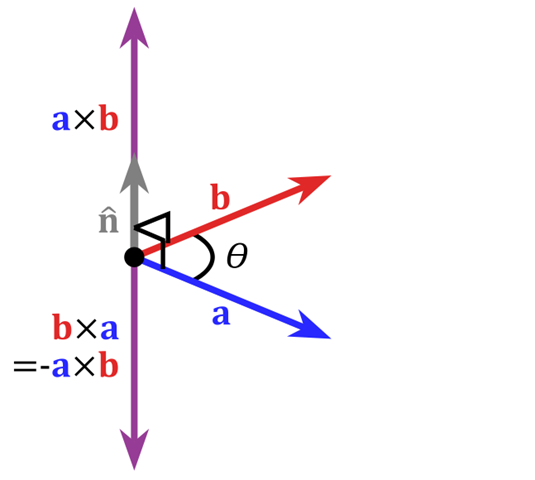对于平面法向量计算实质就是：平面内两非平行向量的叉积(又称"外积、向量积、叉乘")为该平面的法向量。
根据两向量相乘的前后顺序计算得到两个方向相反的法向量。用公式表示为：
平面内两非平行法向量：a, b (c为法向量)
a×b = c
b×a = -c
上式合并即为：
a×b = -b×a
可见，同样是两个向量相乘，不同相乘顺序得到的向量方向是相反的。

另外，平面内两非平行向量的叉乘得到的法向量方向判断方法为：
a×b的方向：右手四指同a方向一致，手掌不动，四手指逐渐弯向b靠近(无法弯向b？请自然的180翻转您的右手再试)，拇指的指向就是a×b的方向，垂直于a和b所在的平面
b×a的方向：右手四指同b方向一致，手掌不动，四手指逐渐弯向a靠近，拇指的指向就是b×a的方向，垂直于b和a所在的平面
a×b的方向与b×a的方向是相反的，且有：a×b=-b×a

因此，在OpenGL中计算三角(四角)面片的法向量时，选取好两个非平行向量后，要得到正确的法向量(主要是方向正确)，一定得注意两个向量相乘的顺序。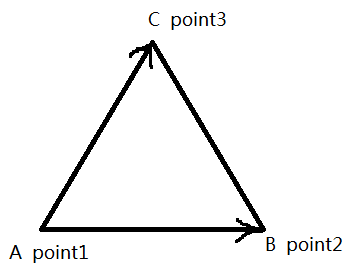向量AC、AB，

AB×AC = 朝向屏幕外的法向量；

AC×AB = 朝向屏幕内的法向量；

如果point1～3属于3维空间，则具体计算步骤为：

用a表示向量AB，用b表示向量AC，则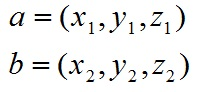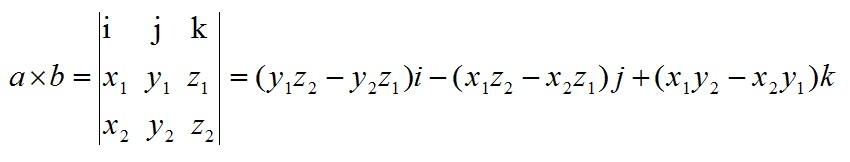其中，i、j、k为3维空间各维度的单位向量。

实战举例

相同的模型、环境光等条件，两个相反的法向量计算方式得到的模型效果如下：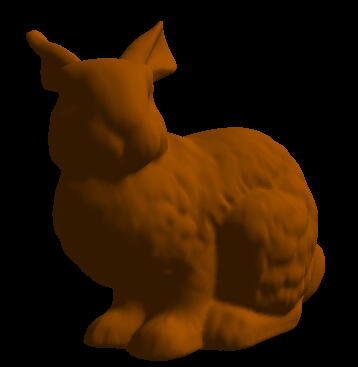顶点法向量

顶点法向量介绍：

顶点法向量：以此点为顶点的所有三角(四角)形的法向量之和即为顶点法向量。

以常见的OBJ文件的3D模型提供了所有顶点的法向量。

总结

1. 以常见的OBJ文件的3D模型提供了所有顶点的法向量，但未提供面的法向量，因此需要自己编写代码实现面的法向量计算。

2. 在OpenGL中为了加快计算速度，一定会将平面法向量和顶点法向量进行“向量归一化”处理。OpenGL顶点法向量归一化后可以加快计算速度。

衍生概念

向量归一化：

向量各分量之和等于1。归一化方法比较简单，因为非归一化的向量之和不一定是1，所以要将向量分别除以这几个向量之和，重新得出的数就是权重向量。比如：

你得到的向量为（0.68, 0.22, 0.09），它们的和是0.99，并不是1，所以要对其进行归一化处理。分别用
0.68/0.99 ；
0.22/0.99 ；
0.09/0.99 ；
然后四舍五入，最后得出的数为（0.6854 0.2213 0.0933），这些数值的和为1，所以叫归一化处理。

归一化的用处：是为了后面数据处理的方便，其次是保证程序运行时加快(各分量变小了)。因为归一化仍然保留着向量各分量的原有权重、特征。所以不会改变向量的原有特性，从而不会影响归一化前后的结果。

参考：

https://blog.csdn.net/nobleman__/article/details/79618331

https://blog.csdn.net/fisherwan/article/details/40897939


展开全文• 要将tensor1后两维构成k个矩阵与tensor2中矩阵做矩阵乘法得到 shape 为[k, m, p]的向量   解决办法： 1，我们知道TensorFlowmatmul已经支持了batch，即： A = tf.Variable(tf.random_normal(...
当tensor1 的 shape 为[k, m, n]， tensor2 的 shape 为 [n, p]时，

要将tensor1的后两维构成的k个矩阵与tensor2中的矩阵做矩阵乘法得到 shape 为[k, m, p]的向量

解决办法：

1，我们知道TensorFlow的matmul已经支持了batch，即：

A = tf.Variable(tf.random_normal(shape=(a, b, n, m)))
B = tf.Variable(tf.random_normal(shape=(a, b, m, k)))
tf.matmul(A, B)

会返回(a,b,n,k)，前面的N个维度会被保留。但是适用情景与题目不符。

2. 我们可以先将用不到的维度通过reshape乘进去，然后有matmul得到结果之后再reshape回来，即

#A.shape() = (k,m,n), B.shape() = (n,p)

reshape_A = tf.reshape(A, [k*m,n])

re = tf.reshape( tf.matmul(reshape_A, B), [k, m, p])

对于形如[k, n, m] 和 [p, n] 的同理，将shape为[k,n,m]通过reshape变为[k*m,n]

如果不是矩阵乘法而是其他操作这种操作是否合理博主还没有进行证明，希望高手能给予指点。

参考：https://www.cnblogs.com/ldzhangyx/p/8076522.html


展开全文tensorflow 矩阵运算
• 名称：矩阵相乘的两种方法 说明：最近，在复习线性代数的时候，老师说到矩阵A*B，可以把B转化为一个个列向量b1，b2，b3分别与A矩阵相乘。这和我以前的想法不同，以前的想法都是按照标准公式A的第一行*B的第一列，...
///
/*
名称：矩阵相乘的两种方法
说明：最近，在复习线性代数的时候，老师说到矩阵A*B，可以把B转化为一个个列向量b1，b2，b3分别与A矩阵相乘。这和我以前的想法不同，以前的想法都是按照标准公式A的第一行*B的第一列，然后相加。今天所认识的，虽然在计算机上计算过程并没有太大的区别，但在线性代数上可以更好的理解什么是线性变换（虽然我现在理解的还不是很深刻）。于是，用一个普通的C++程序实现，算是加强印象吧。
这两种方法我分别把他们叫做行相乘，列相乘算法。
如下图所示：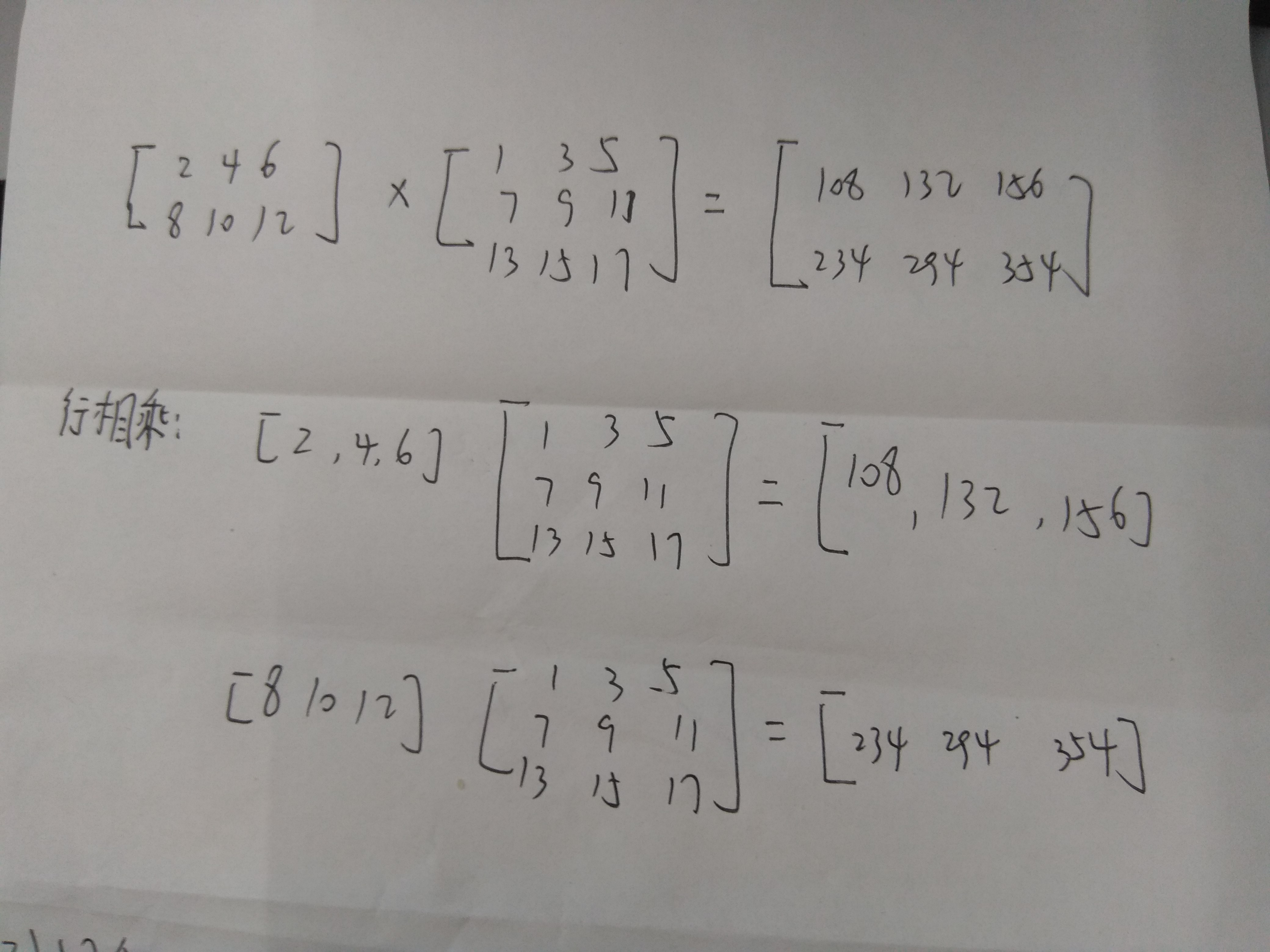![列相乘](https://img-blog.csdn.net/20170626204749777?watermark/2/text/aHR0cDovL2Jsb2cuY3Nkbi5uZXQvcGxtMTk5NTEzMTAw/font/5a6L5L2T/fontsize/400/fill/I0JBQkFCMA==/dissolve/70/gravity/SouthEast)

此种两种算法还可以应用于并行算法中，有时间可以实现以下。


*/
//

#include<iostream>
using namespace std;

class MultiMatrix
{
public:

/*m代表A矩阵的行数，n代表A矩阵的列数（也代表B矩阵的行数）,p代表（B矩阵的列数）
i控制A矩阵的行数变化，j控制B矩阵的列数变化，k控制A矩阵的列数变化（同时控制B矩阵的行数变化）
*/
void RowMulMatrix(int A[],int B[],int m,int n,int p)
{
int res[m][n];      //保存最终结果
int sum;

//矩阵相乘计算
for(int i = 0;i<m;++i)      //后计算A的行
{
for(int j = 0;j<n;++j)     //先计算B的列
{
sum = 0;
for(int k = 0;k<p;++k)
{
sum = sum + A[i][k]*B[k][j];
}
res[i][j] = sum;
}
}

//输出结果
for(int i = 0;i<m;++i)
{
for(int j = 0;j<n;++j)
{
if(j == 0)
cout<<res[i][j];
else
cout<<" "<<res[i][j];
}
cout<<endl;
}
}

void ColMulMatrix(int A[],int B[],int m,int n,int p)
{

int res[m][n];      //保存最终结果
int sum;

for(int j = 0;j<p;++j)      //后计算B的列
{
for(int i = 0;i<m;++i)      //先计算A的行
{
sum = 0;
for(int k = 0;k<n;++k)
{
sum = sum+A[i][k]*B[k][j];
}

res[i][j] = sum;
}

}

//输出结果
for(int i = 0;i<m;++i)
{
for(int j = 0;j<n;++j)
{
if(j == 0)
cout<<res[i][j];
else
cout<<" "<<res[i][j];
}
cout<<endl;
}
}

};

int main()
{
int A = {2,4,6,
8,10,12};

int B = {1,3,5,
7,9,11,
13,15,17};

//测试
MultiMatrix ex;
//
ex.RowMulMatrix(A,B,2,3,3);
cout<<endl;
ex.ColMulMatrix(A,B,2,3,3);

return 0;

}

展开全文• 文章目录一、CPU上实现向量内积二、GPU下单Block分散归约...向量内积运算在数学上的计算公式为 也就是A和B的向量长度相等，相同索引的元素相乘，然后将乘积结果累加，得到结果C 一、CPU上实现向量内积 #include <std

博主CUDA学习系列汇总传送门（持续更新）：编程语言|CUDA入门

文章目录一、CPU上实现向量内积二、GPU下单Block分散归约向量内积三、单Block低线程归约向量内积四、多block向量内积五、cublas库实现向量内积
本文章为 《GPU编程与优化 大众高性能计算》的读书笔记，例子也都取自书中教程。

向量内积运算中各元素的运算间存在简单联系，即需要累加所有元素乘积结果。
向量内积结果的累加过程称为归约（reduction）
向量内积运算在数学上的计算公式为也就是A和B的向量长度相等，相同索引的元素相乘，然后将乘积结果累加，得到结果C

一、CPU上实现向量内积
#include <stdio.h>
#include <iostream>

const int N = 2048;
/* 一、cpu 向量内积 */
template <typename T>
void dot_cpu(T *a, T *b, T *c, int n)
{
double dTemp = 0;
for (int i=0; i<n; ++i)
{
dTemp += a[i] * b[i];
}
*c = dTemp;
}

int main()
{
float a[N], b[N];
float c = 0;
for(int i=0; i<N; ++i) // 为数组a、b赋值
{
a[i] = i * 1.0;
b[i] = 1.0;
}
dot_cpu(a, b, &c, N);
printf("a * b = %f\n", c);
printf("hello world\n");
}

二、GPU下单Block分散归约向量内积

各线程独立完成所有向量元素的乘积运算，然后将乘积的结果累加到向量内积结果。
thread是私有的，block内的threads要进行数据通信，必须使用共享内存或者全局内存。
共享内存的访存速度比全局快一个量级，所以我们这边采用共享内存。

假设单Block 8 线程求内积， 0-7分别表示各线程内内积结果该方法存在缺陷

单线程访问全局的相邻两个单元，违背访问对齐原则，关于对齐可参考gpu显存（全局内存）在使用时数据对齐的问题
共享存储的访问更容易引起 back conflict
什么是back conflict？关于共享存储器，如果同一个warp的不同线程访问到同一个bank中的数据，就会发生 bank conflict。更多参考CUDA-Memory(存储)和bank-conflict

#include "cuda_runtime.h"
#include "device_launch_parameters.h"
#include <stdio.h>
#include <iostream>

const int N = 2048;

const int threadnum = 32;//开32个线程

/* 二、单block 分散归约 */
template <typename T>
__global__ void dot_gpu_1(T *a, T *b, T *c, int n)
{
__shared__ T tmp[threadnum];
const int nThreadIdX = threadIdx.x; //线程ID索引号
const int nBlockDimX = blockDim.x; // 一个block内开启的线程总数
int nTid = nThreadIdX;
double dTemp = 0.0;
while (nTid < n)
{
dTemp += a[nTid] * b[nTid];
nTid += nBlockDimX;
}
tmp[nThreadIdX] = dTemp; // 将每个线程中的内积放入到共享内存中
__syncthreads(); // 同步操作，即等所有线程内上面的操作都执行完

int i=2, j=1;
while(i <= threadnum)
{
if (nThreadIdX%i == 0)
{
tmp[nThreadIdX] += tmp[nThreadIdX + j];
}
__syncthreads();
i *= 2;
j *= 2;
}
if (0 == nThreadIdX)
{
c = tmp;
}
}

int main()
{
float a[N], b[N];
float c = 0;
for(int i=0; i<N; ++i) // 为数组a、b赋值
{
a[i] = i * 1.0;
b[i] = 1.0;
}
float *d_a = NULL, *d_b = NULL, *d_c = NULL;
cudaMalloc(&d_a, N *sizeof(float));
cudaMemcpy(d_a, a, N *sizeof(float), cudaMemcpyHostToDevice);

cudaMalloc(&d_b, N *sizeof(float));
cudaMemcpy(d_b, b, N *sizeof(float), cudaMemcpyHostToDevice);

cudaMalloc(&d_c, sizeof(float));
dot_gpu_1<<<1, threadnum>>>(d_a, d_b, d_c, N);
cudaMemcpy(&c, d_c, sizeof(float), cudaMemcpyDeviceToHost);
std::cout << c << std::endl;
cudaFree(d_a);
cudaFree(d_b);
cudaFree(d_c);
printf("hello world\n");
return 0;
}


三、单Block低线程归约向量内积在归约时，访问共享存储区时，不同线程尽可能少的访问同一个bank中的数据地址。
一块共享存储取中存在多个bank，访问共享内存中的数据时，尽可能离远点。#include "cuda_runtime.h"
#include "device_launch_parameters.h"
#include <stdio.h>
#include <iostream>

const int N = 2048;

const int threadnum = 32;//开32个线程

/* 三、单block 低线程归约向量内积*/
template <typename T>
__global__ void dot_gpu_2(T *a, T *b, T *c, int n)
{
__shared__ T tmp[threadnum];
const int nThreadIdX = threadIdx.x; //线程ID索引号
const int nBlockDimX = blockDim.x; // 一个block内开启的线程总数
int nTid = nThreadIdX;
double dTemp = 0.0;
while (nTid < n)
{
dTemp += a[nTid] * b[nTid];
nTid += nBlockDimX;
}
tmp[nThreadIdX] = dTemp; // 将每个线程中的内积放入到共享内存中
__syncthreads(); // 同步操作，即等所有线程内上面的操作都执行完

int i = threadnum / 2;
while(i != 0)
{
if (nThreadIdX < i)
{
tmp[nThreadIdX] += tmp[nThreadIdX + i];
}
__syncthreads();// 同步操作，即等所有线程内上面的操作都执行完
i /= 2;
}
if (0 == nThreadIdX)
{
c = tmp;
}
}

int main()
{
float a[N], b[N];
float c = 0;
for(int i=0; i<N; ++i) // 为数组a、b赋值
{
a[i] = i * 1.0;
b[i] = 1.0;
}
float *d_a = NULL, *d_b = NULL, *d_c = NULL;
cudaMalloc(&d_a, N *sizeof(float));
cudaMemcpy(d_a, a, N *sizeof(float), cudaMemcpyHostToDevice);

cudaMalloc(&d_b, N *sizeof(float));
cudaMemcpy(d_b, b, N *sizeof(float), cudaMemcpyHostToDevice);

cudaMalloc(&d_c, sizeof(float));
dot_gpu_2<<<1, threadnum>>>(d_a, d_b, d_c, N);
cudaMemcpy(&c, d_c, sizeof(float), cudaMemcpyDeviceToHost);
std::cout << c << std::endl;
cudaFree(d_a);
cudaFree(d_b);
cudaFree(d_c);
printf("hello world\n");
return 0;
}


四、多block向量内积
只有一个block，显然无法发挥GPU的真实性能。
这一节使用多block，block内部采用低线程归约的方法，每个block计算得到一个中间结果并组成一个数组，然后再将中间结果进行二次归约，二次归约可以在cpu上实现，也可以在Gpu上实现，这里只记录在cpu上实现的方法。
#include "cuda_runtime.h"
#include "device_launch_parameters.h"
#include <stdio.h>
#include <iostream>

const int N = 2048;

const int nBlockNum = 16;//开32个block
const int threadnum = 32;//开32个线程

/* 三、单block 低线程归约向量内积*/

/* 四、多block多线程向量内积 */
template <typename T>
__global__ void dot_gpu_3(T *a, T *b, T *c, int n)
{
__shared__ T aTmp[threadnum];
const int nThreadIdX = threadIdx.x; //线程ID索引号
const int nStep = gridDim.x * blockDim.x; // 跳步的步长，即所有线程的数量
int nTidIdx = blockIdx.x * blockDim.x + threadIdx.x; // 当前线程在全局线程的索引

double dTemp = 0.0;
while (nTidIdx < n)
{
dTemp += a[nTidIdx] * b[nTidIdx];
nTidIdx += nStep;
}
aTmp[nThreadIdX] = dTemp; // 将每个线程中的内积放入到对应block的共享内存中
__syncthreads(); // 同步操作，即等所有线程内上面的操作都执行完

int i = threadnum / 2;
while (i != 0)
{
if(nThreadIdX < i)
{
aTmp[nThreadIdX] += aTmp[nThreadIdX + i];
}
__syncthreads(); // 同步操作，即等所有线程内上面的操作都执行完
i /= 2;
}

if (0 == nThreadIdX)
{
c[blockIdx.x] = aTmp;
}

}

int main()
{
float a[N], b[N];
float c = 0;
for(int i=0; i<N; ++i) // 为数组a、b赋值
{
a[i] = i * 1.0;
b[i] = 1.0;
}
float *d_a = NULL, *d_b = NULL, *d_c = NULL;
cudaMalloc(&d_a, N *sizeof(float));
cudaMemcpy(d_a, a, N *sizeof(float), cudaMemcpyHostToDevice);

cudaMalloc(&d_b, N *sizeof(float));
cudaMemcpy(d_b, b, N *sizeof(float), cudaMemcpyHostToDevice);

cudaMalloc(&d_c, sizeof(float) * nBlockNum);
dot_gpu_3<<< nBlockNum, threadnum >>>(d_a, d_b, d_c, N);
float c_tmp[nBlockNum];
cudaMemcpy(&c_tmp, d_c, nBlockNum * sizeof(float), cudaMemcpyDeviceToHost);
for (int i=0; i < nBlockNum; ++i)
{
c += c_tmp[i];
}
cudaFree(d_a);
cudaFree(d_b);
cudaFree(d_c);
std::cout << c << std::endl;
printf("hello world\n");
return 0;
}


五、cublas库实现向量内积
/* 五、cublas 实现向量内积 */
#include "cuda_runtime.h"
#include "device_launch_parameters.h"
#include <stdio.h>
#include <iostream>
#include "cublas_v2.h"
#include "common.h"
const int N = 2048;

int main()
{
float a[N], b[N];
float c = 0.0;
for(int i = 0; i < N; ++i) // 为数组a、b赋值
{
a[i] = i * 1.0;
b[i] = 1.0;
}
float *d_a = NULL, *d_b = NULL;

cublasHandle_t handle;
cublasCreate_v2(&handle); // 创建句柄
cudaMalloc(&d_a, N *sizeof(float));
cublasSetVector(N, sizeof(float), a, 1, d_a, 1);

cudaMalloc(&d_b, N *sizeof(float));
cublasSetVector(N, sizeof(float), b, 1, d_b, 1);

CHECK(cublasSdot_v2(handle, N, d_a, 1, d_b, 1, &c));

CHECK(cudaFree(d_a));
CHECK(cudaFree(d_b));
cublasDestroy(handle); // 销毁句柄

printf("a * b = %f\n", c);
printf("hello world\n");
return 0;
}


CHECK函数的定义如下
#define CHECK(status)                                                                                                  \
do                                                                                                                 \
{                                                                                                                  \
auto ret = (status);                                                                                           \
if (ret != 0)                                                                                                  \
{                                                                                                              \
std::cout << "Cuda failure: " << ret << std::endl;                                                 \
abort();                                                                                                   \
}                                                                                                              \
} while (0)



展开全文• (1)特征值和特征向量计算时间过长，虽然可以运行出来，但是需要处理大约50分钟，这里使用matlab自带eig函数运算，请问有无高效求全部特征值所组成对角阵和全部特征向量所组成矩阵运算方法？ (2)在求得...matlab 奇异矩阵
• 很简单，就一个，用向量叉积（叉乘）计算多边形面积，有两种方法，比较基础：选取多边形一个顶点，然后用其余点和这个顶点相连，形成一个向量，再将每个向量顺时针或逆时针相乘，这样就能得到整个多边形...
• 1、矩阵的乘法和除法 （1）点乘、点除…是对应元素之间进行运算： （2)两矩阵之间的乘法，直接用*即可，但要求第一个矩阵的列数等于第二个矩阵的行数。...实际的计算公式如下(只是为了便于理解) ...matlab
• 记录最近用到两种方法，根据计算精度要求不同需适当修改。 避免两个大__int64值相乘！ 尽量少使用开方运算可以提高精度。 asin函数求出角是0到90度,需要根据实际情况还原到原来大小。 #pragma ...模板
• GEMM矩阵相乘与深度学习1. GEMM矩阵相乘1.1 GEMM算法基础... GEMM与卷积计算2.1 计算卷积的方法2.2 Img2col2.2.1 CNN中张量存储2.2.2 卷积运算转化为GEMM 1. GEMM矩阵相乘 1.1 GEMM算法基础   GEMM（General Matrix
• 引子：降维打击 科幻小说《三体》里一种很魔幻攻击方法——降维打击，以其神奇作用方式和巨大威力刷新了我们三观。而在矩阵乘法计算中，这种降维打击时刻存在...只要将两个矩阵相乘，就能根据 方法 X 对象...
• 什么是四元数：提到四元数...与向量相乘 计算物体右前方30度，10米远坐标？ 第一种方法： TransformPoint ：自身坐标转世界，本质就是对向量做旋转然后叠加到物体上 第二种方法： this.transform.position...
• 利用系数矩阵的对称性和分块Toeplitz型矩阵与向量相乘的快速算法，解决了系数矩阵的存储和计算问题，导致空间域位场延拓成为可能，为研究新的位场延拓方法和分析模型的扩展误差提供了一个新的途径。利用模型数据和...研究论文
• 文章目录@[toc]1、并行方法------矩阵与向量的相乘运算1.1、Rowwise Block-striped1.2、Columnwise Block-striped1.3、Checkerboard Block Decomposition2、并行方法------矩阵与矩阵的相乘运算2.1、Block- Striped ...
• 向量化编程实现 Vectorized implementation 一向量化编程 Vectorization ...为了提升特定数值运算操作（如矩阵相乘、矩阵相加、矩阵-向量乘法等）速度，数值计算和并行计算的研究人员已经努力了几十年。...
• 上一节讲解了矩阵初等变换，本章将学习并了解向量。此章请认真学习。 向量 ...（2）内积：两个向量α，β内积，用记号（α，β）表示，计算方法为：对应位置元素相乘并相加； （α，β）...
• 作为例子问题是1000×10001000 \times 10001000×1000矩阵和向量相乘。使用了4个线程。 先打一下预防针。矩阵乘向量这个问题太简单了，一定要使用Foster设计方法反而觉得Foster有些累赘。例如信息传递、整合实际上...
• 在深度学习中，神经网络将权重储存在矩阵当中，基于线性代数的运算在 GPU上，可以对矩阵进行简单迅捷的计算处理。 小规模的矩阵运算，可以用 for 循环来快速运算。但是一旦遇到巨大的数据量，循环语句就会拖慢...pytorch 深度学习 机器学习 人工智能
• 法一：从一个例题出发来看...以此类推，写出整个矩阵，与x1向量相乘，即得结果。计算过程完全符合公式。 法2:上面的方法计算起来速度不够快，我们可以通过一个简单的方法快速写出x2矩阵。按列来观察，第一列..
• 之前有个需求，就是比较2个文本是否相近。...最终发现，如果是文本计算特征值，把文本字符串输入后，简单简化为每个char自相乘，再相加 = 向量的平方。例如：HI = (char 72)(char 73).e...
• 利用系数矩阵的对称性和分块Toeplitz型矩阵与向量相乘的快速算法，解决了系数矩阵的存储和计算问题，使得 空间域位场延拓成为可能，为研究新的位场延拓方法和分析延拓误差提供了一条新的途径．利用模型数据和...研究论文
• 很多应用的计算最终都转化为矩阵相乘来完成，例如推荐算法中的协同过滤就可以套上去。协同过滤（collaborative filtering）是推荐系统中一类广泛使用的方法，协同过滤中两种比较出名的方法item-based CF、user-...solr 矩阵运算 协同过滤 lucene
• 向量的点乘,也叫向量的内积、数量积，对两个向量执行点乘运算，就是对这两个向量对应位一一相乘之后求和操作，点乘结果是一个标量。 向量 向量 则a,b点乘 点乘几何意义是可以用来表征或计算两个向量之间...
• 范例1-13 字符串长度的计算 28 ∷相关函数：strlen函数 1.1.14 字符串的复制 29 范例1-14 字符串的复制 29 ∷相关函数：strcpy函数 1.1.15 字符串的替换 31 范例1-15 字符串的替换 31 ∷相关函数：strrep函数...
• 针对一套混凝土细观力学分析程序,在分析其计算方法与计算效率不足之后,提出了采用稀疏矩阵与稀疏向量技术来高效实现有限元刚度矩阵装配过程算法,并采用双门槛不完全 Cholesky分解预条件技术与CG法相结合来高效地...
• 对伪逆矩阵进行因式分解以求解正规方程： ... 分解后结果存储在 object 中，稍后可用于与任何目标空间向量 (RHS) 相乘。 灵感来自 FACTORIZE http://www.mathworks.com/matlabcentral/fileexchange/24119matlab
• 6.6.8 实例：一维矩阵与向量相乘 6.6.9 实例：单源最短路径 6.6.10 实例：样本排序 6.7 进程组和通信器 6.8 书目评注 习题 第7章 共享地址空间平台编程 7.1 线程基础 7.2 为什么要用线程 7.3 POSIX线程...
• 首先计算摄像机视锥体四条射线向量进行线性插值，插值后值便是该像素在世界空间坐标下到摄像机方向。 然后通过与深度值相乘即可得到摄像机位置到该像素的向量，加上摄像机位置则是该像素在世界空间中...
• 方法来源于推荐系统中矩阵分解，通过最小化目标函数来估计单词向量，如果两个单词向量越接近，则这两个单词后面接某个单词概率应相似，通过h向量和v向量相乘计算连续语言模型中表格中值。 连续语言模型和...